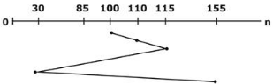# GATE Solved Paper 2020 - GATE 2020

• A

Neither Rule 1 nor Rule 2 is L-attributed• B

Both Rule 1 and Rule 2 are L-attributed• C

Only Rule 1 is L-attributed.• D

Only Rule 2 is L-attributed.• Option : C
• Explanation :
Rule 1: Rule 1: It is Rule 1: L-attributed by definition.
Rule 2: Rule 2: It is not L-attributed because this expression (X. Rule 2: i = A.i + Y.s) fails to satisfy the definition of L-attributed.

 CODE SECTION P
wait(a); count=count+1;
if (count==n) signal(b);
signal(a); wait(b); signal(b);
 CODE SECTION Q

What does the code achieve?

• Option : C
• Explanation :
Subject Operating Systems
a = 1 b = 0 count = 0
Code section P: For process 1
Wait (a) ⇒ a = 0
Count ++ ⇒ count = 1
If (count = = n) ⇒ false
signal (a) ⇒ a = 0
wait (b) process blocked
As shown above, all processes execute wait (b) before, signal (b) in if condition.
Because, ‘if’ condition becomes True only for process Pn(nth process) [∵ if (count = = n)]
So, before Pn process finishes the code section, P, no other process executes code section Q.
Hence, no process executes code section Q before every process has finished code section P.

• A

Θ(E∣ log ∣V∣)• B

Θ(∣E∣ + ∣V∣)• C

Θ(∣E∣.∣V∣)• D

Θ(∣V∣)• Option : D
• Explanation :
G = (V, E) T = MST
Weighted edge ( μ, v) is added to T.
To verify it is still a MST or not, we need to compare with all the vertices. So, it would be O(V).

• A

L1 is regular and L2 is context-free.• B

L1 is context-free but not regular and L2 is context-free• C

L1 is context-free but L2 is not context-free.• D

Neither L1 nor L2 is context-free.• Option : A
• Explanation :
L1 is regular and L2 is CFL.
L1: {ω xyx| ω, x, y ϵ (0 + 1)*}
We can write the regular expression for this,
Let, x = 0
L11 = ω 0 Y 0 ⇒ ( 0 + 1)+ 0(0 + 1)+0
x = 1
L12 = ω 1 Y 1 ⇒ we can generate all the strings and since a regular expression can be written for 4, we can say 4 is regular.
L2 is CFL:
L2 = {xy| x,y ϵ (a + b)*, |x| = |y|, x ≠ y}
L2 generates strings of even length, which can be done by PDA, therefore L2 is CFL.

• A

T is serviced before P.• B

The head reverses its direction of movement between servicing of Q and P• C

R is serviced before P.• D

Q is serviced after S, but before T.• Option : D
• Explanation : Given P : 155
Q : 85
R : 110
S : 30
T : 115
Current head position = 100
SSTF = algorithmFrom the above sequence, we can say that option D is correct.
Related Quiz.
GATE 2020